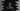# C++ program to find the area of a square using three different methods## Introduction :

In this post, we will learn how to find the area of a square using three different methods in C++. Our program will take the length of one side of the square and it will calculate the result. The formula to calculate square area is simple. It is side * side where side is the length of one side. Let’s have a look at the programs :

### Method 1: C++ program to calculate the area of a square :

``````#include <iostream>
using namespace std;

int main()
{
int side, area;

cout << "Enter the side length : " << endl;
cin >> side;

area = side * side;
cout << "Area of the square is : " << area << endl;
}``````

Here, we are asking the user to enter the side length. Using cin we are storing that value in the variable side. We have two variables here. One is to hold the length of side and the another is to hold the area. Once we get the side length, we are calculating the area and storing that value in the variable area. In the last line, we are printing out that value.

Following are some sample outputs of this program :

``````Enter the length of side :
24
Area of the square is : 576

Enter the length of side :
123
Area of the square is : 15129``````

### Example 2: C++ program to calculate the area of a square using function :

A function is used to separate out common code in a program. For example, if you have one common process to calculate something, you can put that logic in a function and invoke that function from anywhere you want. Optionally, a function can take values as its parameter and it can return a value to the caller. In our case, the function will take the side of the square as the parameter and it will return the area of the square. Below is the complete C++ program :

``````#include <iostream>
using namespace std;

int findArea(int length){
return length * length;
}
int main()
{
int side, area;

cout << "Enter the length of side : " << endl;
cin >> side;

cout << "Area of the square is : " << findArea(side) << endl;
}``````

Here, findArea is a function to calculate the area. It takes the square side length as parameter and returns the area of the square. It will give similar output as the previous example.

### Method 3: Using pow function to find the area of a square in C++ :

pow(base, exponent)_ function is defined cmath header of C++. We can use it to calculate the area of a square. It returns base to the power exponent. For exponent = 2, it will return the square of a number. Example program :

``````#include <iostream>
#include <cmath>
using namespace std;

int findArea(int length){
return pow(length, 2);
}

int main()
{
int side, area;

cout << "Enter the length of side : " << endl;
cin >> side;

cout << "Area of the square is : " << findArea(side) << endl;
}``````

Make sure to include cmath in the function. It will give similar output.